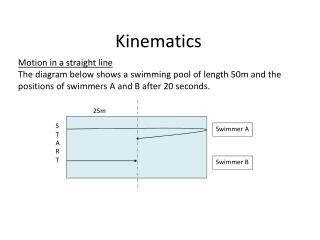DownloadDownload PresentationKinematics

# Kinematics

Télécharger la présentation## Kinematics

- - - - - - - - - - - - - - - - - - - - - - - - - - - E N D - - - - - - - - - - - - - - - - - - - - - - - - - - -
##### Presentation Transcript

1. 25m START Swimmer A Swimmer B • Motion in a straight line • The diagram below shows a swimming pool of length 50m and the positions of swimmers A and B after 20 seconds. Kinematics

2. D T S Definition Distance from a given position with an associated sign is called Displacement. Units are always m. Definition The Velocity of a body has a magnitude (size) and an associated sign. Units are always m/s or written ms-1 The above workings make use of the following triangle of formulae for Distance, speed, time.

3. Acceleration 3m/s 13m/s A B In the diagram above let’s say that a ball moves from A to B such that its velocity at A is 3m/s and its velocity at B is 13m/s. Furthermore the time taken to travel from A to B is 5 seconds. Here we say that the ball has accelerated whilst travelling from A to B.

4. 30m/s 6m/s A B In the diagram above let’s say that a ball moves from A to B such that its velocity at A is 30m/s and its velocity at B is 6m/s. Furthermore the time taken to travel from A to B is 6 seconds. Here we say that the ball has decelerated whilst travelling from A to B.

5. Here we can write down the formula for acceleration as Acceleration = Here we have the basics of the formulae required in kinematics a um/s vm/s A B t t=0 s

6. With u = the initial velocity v = the final velocity t = time taken s = displacement a = acceleration (constant) Then we may use the following formulae to solve any number of problems!

7. Example A car accelerates from a velocity of 16 m/s to a velocity of 40m/s in a distance of 500m. Calculate the acceleration of the car.

8. Example Initially, a particle P is moving with uniform acceleration 6m/s2 has a velocity at the point O of 3m/s. Find its velocity after 2s and its displacement from O after 4s.

9. Example • A body moves along a straight line from A to B with Uniform acceleration  m/s2. The time taken is 12 seconds and the velocity at B is 25 m/s. • Find • The velocity at A • The distance AB.

10. Example The displacement from its original position of a particle moving with uniform acceleration is 6m after 2s and 20m after 4s. Find the displacement 6s after the start of the motion.

11. Example A car decelerates from a velocity of 36m/s. The magnitude of the deceleration is 3m/s2. Calculate the time required to cover a distance of 162m.

12. Example • A stone slides in a straight line across a horizontal sheet of ice. It passes point A with velocity 14m/s, and point B 2 seconds later where AB = 30. Retardation is uniform. • Calculate • The velocity at B • The retardation • The time and displacement from A at which the stone comes to rest.